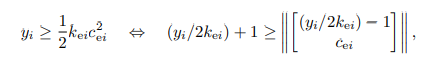# Numerical problems / Inaccurate solution for a SOCP

Dear CVX community, maybe you could share your thoughts about a possible source of numerical problems in a SOCP with a lot of conical constraints. It is a structural equilibrium problem for cable networks akin the one formulated by Kanno*.
The solution of the primar problem is not unique. There is a part of network where cable are in the slack state and have zerro stiffness thus having perfect freedom of motion.

*Kanno, Y., & Ohsaki, M. (2006). Contact analysis of cable networks by using second-order cone programming. SIAM Journal on Scientific Computing, 27(6), 2032-2052.

The cvx sequence running the problem is
cvx_begin
variable xj(2nj)
variable ca(na) nonnegative
variable ya(na) nonnegative
minimize(sum(ya));
subject to
for a=1:na
ca(a) >= norm(Bmat([2
a-1,2a],xj)-La(a);
ya(a)/2+1 >= norm( [ ya(a)/2-1;ca(a)] );
end
%BCs
xj(2
jD1-1) == xjbar(2
jD1-1);
xj(2jD1) == xjbar(2jD1);
xj(2jD2-1) == xjbar(2jD2-1);
xj(2jD2) == xjbar(2jD2);
cvx_end

The typical outcome from cvx solver

I doubt anyone can tell you why SeDuMi cannot solve your problem to the requested accuracy.

When I read network then I think maybe your constraint matrix is not of full rank. That could be one among many problems causing a hard time for SeDuMi.

You could try Mosek or SDPT3 instead. If they also experience problems, then most likely your problem is nasty.

If one of them works well, then you have the solution you need.

Thank you for a prompt reply. Neither Mosek nor SDPT3 show any improvement. They all experience similar problems reaching high accuracy.
The Bmat matrix in conical constraints has rank 2 deficience ( just the rigid body motios in 2D ) which anyway should be compensated by the displacement BCs. Is there any sensible way to test this part of the problem set up?

You have proved your problem is numerically tough.

At least for me, it is not easy to say why since it requires a full understanding of the problem.

Note 1:

This

ya(a)/2+1 >= norm( [ ya(a)/2-1;ca(a)] );

looks fishy because it is fairly similar to

x >= norm([x;1])

This is clearly infeasible but as x tends to infinity it is getting closer to feasibility.
So is illposed.

Note 2:

An idea

||Bx|| = ||QRx|| = ||Rx||

where Q is orthogonal. So you might replace B with R where QR is a QR factorization B.

Thank you for great insights!

Re Note 1: this conical constraint is just a way to introduce a quadratic term to the objective. and it is feasible, cause there’s +1 on lhs and -1 on rhs
It’s a trick I copycat from Kanno (2011)But this is irrelevant. Because, I have the same problem in other formulations that do not involve this type of inequaity. Anyway, CVX can digest quadratic objectives. Which I also checked out.

Re Note 2: I may look into it. The problem is that a have a LOT of low-dimensional conical constraints that only involve a few variables. I have one SOC for each memeber of the network. Thus it only involves a submatrix of Bmat every time. Need to think if decomposition will help the matter on this scale.

This sequence solves the same primal problem without the conversion of the quadratic objective with the help of an additional variable y.

``````cvx_begin
variable xj(2*nj)
variable ca(na) nonnegative
minimize(sum(1/2*(ca.^2)));
subject to
for a=1:na
ca(a)>=norm(Bmat([2*a-1,2*a],:)*xj)-La(a);
end
%BCs
xj(2*jD1-1) == xjbar(2*jD1-1);
xj(2*jD1) == xjbar(2*jD1);
xj(2*jD2-1) == xjbar(2*jD2-1);
xj(2*jD2) == xjbar(2*jD2);
``````

cvx_end

I would do

minimize norm(ca)

and not the sum of squares.

In any case

ya(a)/2+1 >= norm( [ ya(a)/2-1;ca(a)] );

is from a theoretical point of view bad i.e. it will increase the number of iterations compared to the revised formulation.

The reason is that the constraint ultimately leads to the introduction of 1 conic quadratic constraint for each a. Your revised problem or my suggestion above requires only one conic quadratic constraint.

I curious as to why you initially wrote the problem with the unneeded ya(a) variables.

1 Like

1. The motivation behind introducing extra ya variables comes from Kanno, who suggested this transformation of the original problem to convert it into a classical SOCP with linear objective.

2. The suggested formulation with vector norm norm(ca) objective dispayed no essential advantages with respect to the numerical performance.

3. This primal problem is known not to have a unique solution. There is an entire multidimentional face/edge on the boundary of the feasible domain where equivalent optima are located. My judgement is that to be a source of ill conditioning. Among all the other solvers Mosek seems to handle this ill-conditioning the best (apparently it has better algebra for defining the search direction, or maybe their manipulations with conical constraints do the trick).

4. Anyway, an alternative dual formulation (a complementary energy principle instead of the potential energy principle) to the contrary has a unique solution. But Sedumi runs into numerical problems with that too once the solution gets closer to the constraints (again, it is expected to be placed on the apexes of many of the cones). Mosek seems to handle this situation more or less satisfactory.

cvx_begin
variable tj(2nj)
variable qa(2
na)
variable va(na) nonnegative

`````` minimize( sum(  va+1/2*(va.^2)./La ) - dot(tj,xjbar) );
subject to

for a=1:na
va(a) >= norm(qa([2*a-1,2*a]));
end
tj([2*jN-1,2*jN]) == 0;
tj == Bmat'*qa;
``````

cvx_end